• 第7.24节 Python案例详解：使用property函数定义属性简化属性访问代码实现 一、 案例说明 本节将通过一个案例介绍怎么使用property定义快捷的属性访问。案例中使用Rectangle类： 1、 在类内定义了两个私有属性长度...
第7.24节 Python案例详解：使用property函数定义属性简化属性访问代码实现

一、    案例说明
本节将通过一个案例介绍怎么使用property定义快捷的属性访问。案例中使用Rectangle类：
1、    在类内定义了两个私有属性长度和宽度self.__length、self.__width；
2、    定义了这两个属性的get方法getLen、getWidth；
3、    定义了一次返回这两个属性的get方法getSize，返回一个由长度和宽带值组成的元组，并为了跟踪执行轨迹在方法中输出了痕迹；
4、    定义了self.__length、self.__width的set方法setLen、setWidth，并为了跟踪执行轨迹在方法中输出了痕迹;
5、    定义了删除两个属性的方法delSize
6、    使用property分别将相关操作绑定了size、len、width属性，size支撑get、set和del，其他两个属性不支持del

size = property(getSize,setSize,delSize,'用元组存储的长方形的长和宽')
len = property(getLen,setLen,0,'长方形的长') #虽然执行效果一样，但参数中的0最好用None
width = property(getWidth,setWidth,0,'长方形的宽')

二、    案例代码(交互方式下逐句执行)

class Rectangle():
def __init__(self,length,width): self.__width,self.__length = width,length
def setSize(self,size):
print("execute setSize")
self.__length,self.__width=size #使用序列解包获取长度和宽度
def getSize(self):
print("execute getSize ")
return self.__length,self.__width
def delSize(self):
print("execute delSize ")
self.setSize((0,0))
def setLen(self,length):
print("execute setLen")
self.__length=length
def getLen(self):
print("execute getLen")
return self.__length
def setWidth(self,width):
print("execute setWidth")
self.__width=width
def getWidth(self):
print("execute getWidth")
return self.__width

size = property(getSize,setSize,delSize,'用元组存储的长方形的长和宽')
len = property(getLen,setLen,0,'长方形的长')
width = property(getWidth,setWidth,0,'长方形的宽')

rect = Rectangle(5,3)
rect.size,rect.len,rect.width
rect.size=(5,4)
rect.size,rect.len,rect.width
help(Rectangle.size)
print(Rectangle.len.__doc__,Rectangle.width.__doc__,sep='\n')
del rect.size

三、    案例截屏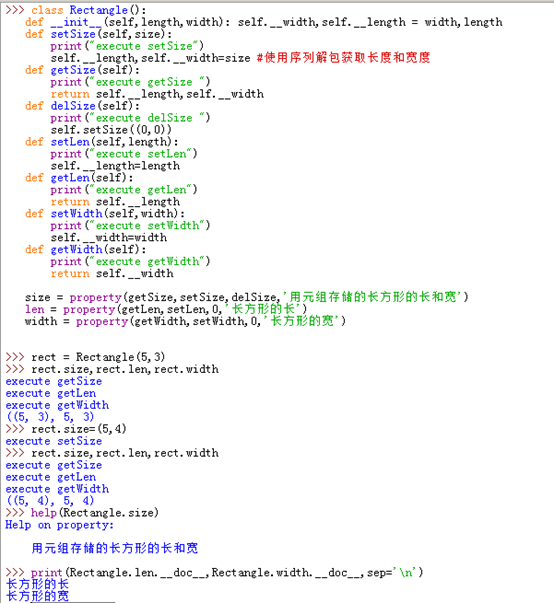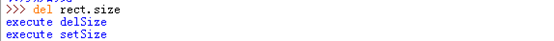从上面的轨迹可以清晰看到，所有对property定义属性的访问都调用了对应的访问方法，赋值并不是直接赋值，而是调用setSize、setLen、setWidth方法，del并不是直接删除，而是调用delSize方法，查询变量则是调用的get方法。
本节结合案例详细介绍了对在类中使用property函数定义一个可供外部简便访问的实例属性的详细过程，通过案例可以明确地看到property定义的属性的读写访问都会通过内部的方法进行，并且属性与内部实例变量之间可以是一对多的关系，甚至是不和实例变量建立关系直接执行方法（如把上面相关的方法访问实例变量的语句去掉只留下print语句就是这样的效果）。
老猿Python（https://blog.csdn.net/LaoYuanPython）系列文章用于逐步介绍老猿学习Python后总结的学习经验，这些经验有助于没有接触过Python的程序员可以很容易地进入Python的世界。
欢迎大家批评指正，谢谢大家关注！

展开全文property
• 第7.25节 Python案例详解：使用property函数定义与实例变量同名的属性会怎样？ 一、 案例说明 我们上节提到了，使用property函数定义的属性不要与类内已经定义的普通实例变量重名，如果是重名会发生什么呢？本...
第7.25节 Python案例详解：使用property函数定义与实例变量同名的属性会怎样？

一、    案例说明
我们上节提到了，使用property函数定义的属性不要与类内已经定义的普通实例变量重名，如果是重名会发生什么呢？本案例通过这个例子一是说明重名可能产生的后果，也想说明property定义属性在类内访问会遇到什么情况。
为了直接说明问题，本案例还是以上节的Rectangle为例，不过去掉了大部分属性和方法，只留下了可以说明问题的必要代码。
二、    案例代码
1.    定义类，类体内定义了实例变量self.length，并定义了类的构造方法、setLen、getLen方法；

class Rectangle():
def __init__(self,length,width): self.width,self.length = width,length
def setLen(self,length):
print("execute setLen")
self.length=length
def getLen(self):
print("execute getLen")
return self.length

2.    通过property定义属性length，这个属性被设置成可读写的，与实例变量self.length变量名相同；

length = property(getLen, setLen,None,'长方形的长')

3.    定义实例对象

rect = Rectangle(5,3)

执行后，我们看到系统不停的输出“execute setLen”，重复很多次后报“RecursionError: maximum recursion depth exceeded while pickling an object”。部分执行截图：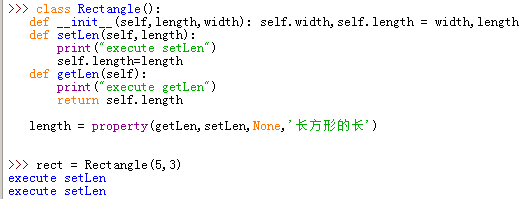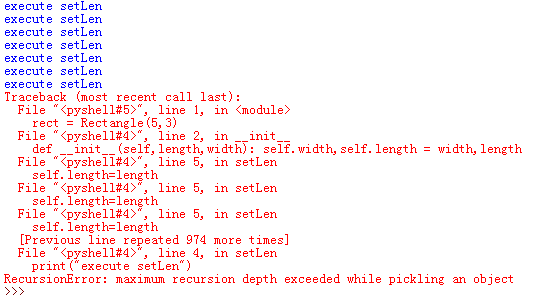三、    案例问题分析
1.    原因分析
对这个问题老猿初略分析原因如下：
1)    从上述报错信息可以看出，报错是因为定义实例变量执行构造函数对实例变量self.length赋值时触发了setLen方法，而setLen又会执行self.length赋值导致setLen不停递归调用，最终导致资源耗尽才终止；
2)    为什么self.length的赋值会触发setLen调用呢，肯定是因为自定义属性与实例变量同名导致的。但为什么会这样老猿没有明白，详见下面老猿的疑惑。
2.    老猿的疑惑
为什么定义属性与实例变量同名就会触发递归调用？老猿暂时无法深入分析，在这有两个问题老猿没有弄明白：
1)    property定义的实例属性，其定义语句是在类体中执行的，按理来说这个定义的实例属性是类变量，其类型比较特殊，是property类型，但根据这个实例属性的访问情况来看是通过实例访问，并且不同实例对象之间互不干扰的，因此这个实例属性应该不是类变量，这个不知道property是怎么实现的？
2)    第二个问题是property类型主要有什么属性，可以让它和实例变量关联，我们知道它有四个参数，其中三个方法会被property的 getter(), setter()和delete()所使用，但是哪个属性记录了这些方法访问的实例？
本节介绍了使用property定义与实例变量相同的属性，发现这种定义会导致程序触发递归调用而异常退出，这说明：
1、    不能使用property定义与实例变量同名的实例属性；
2、    实例属性定义后任何在类内和类外的访问都会触发相关方法执行，因此在访问该属性时一定要注意，防止出现本例类似的递归调用。
老猿Python（https://blog.csdn.net/LaoYuanPython）系列文章用于逐步介绍老猿学习Python后总结的学习经验，这些经验有助于没有接触过Python的程序员可以很容易地进入Python的世界。
欢迎大家批评指正，谢谢大家关注！

展开全文property
• 接下来我们将使用高尔夫球的案例来写梯度下降的算法. 链接：https://pan.baidu.com/s/1GJ20Kha2AMF5Qw0hRUarVQ  提取码：0fjw  数据集我保存到云盘,需要的可以自己下载一下 #梯度下降 import pandas ...


什么是梯度下降，举个栗子，（假设地面无障碍物）我们在山顶丢一个网球，啪，一下就越过这个坡了，然后在另一个沟里来回跳动，一直到达最低点。那么问题来了，这这里在跳跃的过程中，直接越过第一个坡度。这样的结果就是使得我们的小球无法到达最低点，对应到程序上就是我们在梯度下降的时候无法找到最优值。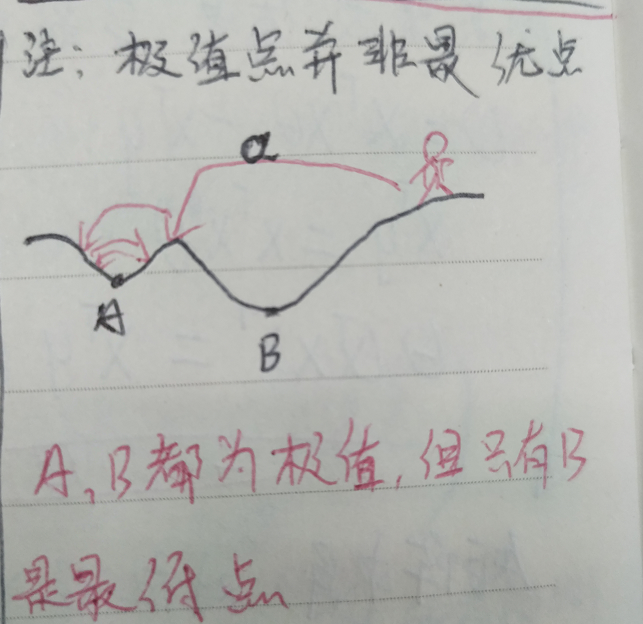梯度下降，就是是在不断就是出于最高点，先下走一个步长，不断的走，或回撤步长的过程。使得我们找到目标的最优值。那么有个问题就是步长（也称为学习率）取值为多少才适合？ 过大的步长容易使得跳过最优值，过小的步长使得梯度下降的效率过低。

梯度下降的过程,其实就是迭代求解的过程.

在公式推导之前，我们先引入预测值函数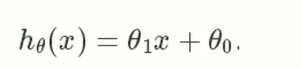hθ(x) :预测值           θ1 :权重                           θ0 :偏置

还有,损失函数（也叫代价函数）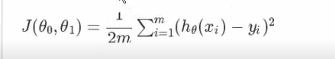该损失函数是由高斯分布的预测误差求取对数拟然函数,结果的被减项得出的.原结果1/2并不是1/2m,这里分母加了m,是为了去除样本对损失函数的影响,是的减少样本数对损失函数的影响. (这里如果为加1/m,结果将是样本次数越多,损失函数越大,样本对损失函数的影响非常大.)

根据损失函数,我们接下来对 权重θ1 与 偏置 θ0 ,求取对应的偏导数.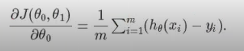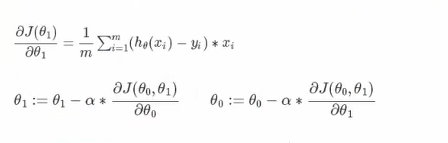上面的  α 就是我们设置的学习率.

接下来我们将使用高尔夫球的案例来写梯度下降的算法.

链接：https://pan.baidu.com/s/1GJ20Kha2AMF5Qw0hRUarVQ
提取码：0fjw
数据集我保存到云盘,需要的可以自己下载一下

#梯度下降
import pandas
import matplotlib.pyplot as plt

# 高尔夫球数据集
pga = pandas.read_csv("pga.csv")

# 数据标准化
pga.distance = (pga.distance - pga.distance.mean()) / pga.distance.std()
pga.accuracy = (pga.accuracy - pga.accuracy.mean()) / pga.accuracy.std()
print(pga.head())

plt.scatter(pga.distance, pga.accuracy)
plt.xlabel('normalized distance')
plt.ylabel('normalized accuracy')
plt.show()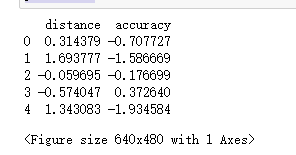##distance: 挥杆之后的距离

## accuracy : 精度

# accuracyi=θ1distancei+θ0+ϵ

from sklearn.linear_model import LinearRegression
import numpy as np

# We can add a dimension to an array by using np.newaxis
print("Shape of the series:", pga.distance.shape)
print("Shape with newaxis:", pga.distance[:, np.newaxis].shape)

# fit()中的X变量必须是二维的
lm = LinearRegression()
lm.fit(pga.distance[:, np.newaxis], pga.accuracy)
theta1 = lm.coef_
print (theta1)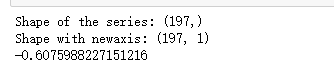# 单变量线性模型的成本函数
# hθ(x) = θ1X + θ0
#计算损失函数,J(θ0,θ1) = 1/2m *累加 (hθ(Xi)-Yi)**2
def cost(theta0, theta1, x, y):
# 初始化成本
J = 0
# 观测次数
m = len(x)
# 遍历每个观测值
for i in range(m):
# 计算假设
h = theta1 * x[i] + theta0
# 累加成本
J += (h - y[i])**2
# 平均和规范成本
J /= (2*m)
return J

# 损失函数 theta0=0 and theta1=1
print(cost(0, 1, pga.distance, pga.accuracy))

theta0 = 100
theta1s = np.linspace(-3,2,100)
costs = []
for theta1 in theta1s:
costs.append(cost(theta0, theta1, pga.distance, pga.accuracy))

plt.plot(theta1s, costs)
plt.show()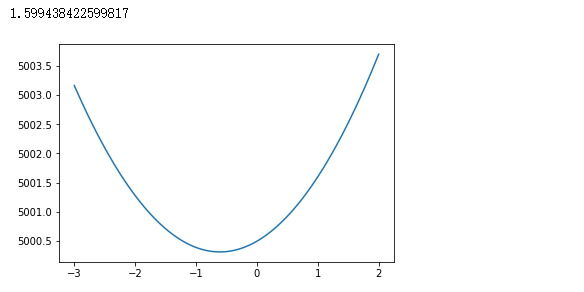import numpy as np
from mpl_toolkits.mplot3d import Axes3D

# 使用Matplotlib的地表绘图示例
# 创建x和y变量
x = np.linspace(-10,10,100)
y = np.linspace(-10,10,100)

# 我们必须创建变量来表示x和y中每一对可能的点
# ie. (-10, 10), (-10, -9.8), ... (0, 0), ... ,(10, 9.8), (10,9.8)
# x和y需要转换成100x100矩阵来表示这些坐标
# np.meshgrid will build a coordinate matrices of x and y
X, Y = np.meshgrid(x,y)
#print(X[:5,:5],"\n",Y[:5,:5])

# 计算一个三维抛物线
Z = X**2 + Y**2

# 打开一个画布
fig = plt.figure()
# I初始化3 d图
ax = fig.gca(projection='3d')
# 绘制图像
ax.plot_surface(X=X,Y=Y,Z=Z)

plt.show()

theta0s = np.linspace(-2,2,100)
theta1s = np.linspace(-2,2, 100)
COST = np.empty(shape=(100,100))
# Meshgrid为参数
T0S, T1S = np.meshgrid(theta0s, theta1s)
# 累计每个参数组合计算成本
for i in range(100):
for j in range(100):
COST[i,j] = cost(T0S[0,i], T1S[j,0], pga.distance, pga.accuracy)

# 绘制3d图
fig2 = plt.figure()
ax = fig2.gca(projection='3d')
ax.plot_surface(X=T0S,Y=T1S,Z=COST)
plt.show()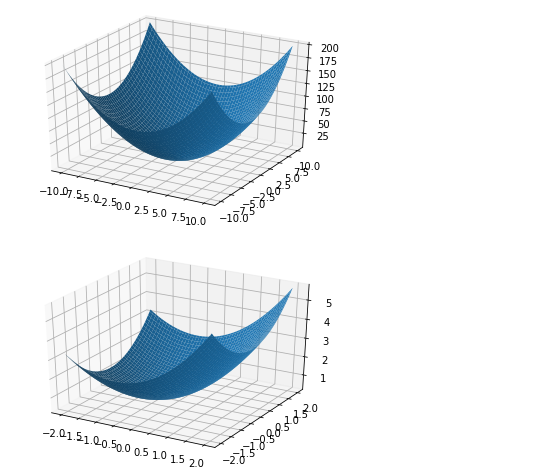#假设函数的权重求导
def partial_cost_theta1(theta0, theta1, x, y):
# 假设函数
h = theta0 + theta1*x
# 对θ1求导后的公式进行计算,θ1的偏导数
diff = (h - y) * x
# 累加偏导数和,除于样本个数
partial = diff.sum() / (x.shape)
return partial

partial1 = partial_cost_theta1(0, 5, pga.distance, pga.accuracy)
print("partial1 =", partial1)

#假设函数的偏置求导
def partial_cost_theta0(theta0, theta1, x, y):
# 假设函数
h = theta0 + theta1*x
# 对θ0求导后的公式进行计算,θ1的偏导数
diff = (h - y)
# 累加偏导数和,除于样本个数
partial = diff.sum() / (x.shape)
return partial

partial0 = partial_cost_theta0(1, 1, pga.distance, pga.accuracy)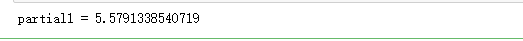# x是特征向量 -- distance
# y 是目标标签 -- accuracy
# alpha 是学习率
# theta0 是偏置
# theta1 是权重
def gradient_descent(x, y, alpha=0.1, theta0=0, theta1=0):
max_epochs = 1000 # 最大迭代次数
counter = 0      # 计数器
c = cost(theta1, theta0, pga.distance, pga.accuracy)  ## 损失函数
costs = [c]     # 储存损失函数
# 设置收敛阈值，找出代价函数在何处最小
# 以前成本与当前成本之差,小于这个值我们就说参数收敛了,即已经快找点目标了
convergence_thres = 0.000001  #收敛的阈值
cprev = c + 10   #这里加10是为了设置一个起始值,要计算起始值与损失函数的距离
theta0s = [theta0]
theta1s = [theta1]

# 当成本收敛或我们遇到大量迭代时，我们将停止更新
while (np.abs(cprev - c) > convergence_thres) and (counter < max_epochs):
cprev = c #这里把损失函数的值赋给了起始值,使得范围进一步缩小
# 学习率乘与对θ求导
update0 = alpha * partial_cost_theta0(theta0, theta1, x, y)
update1 = alpha * partial_cost_theta1(theta0, theta1, x, y)

# 同时更新theta0和theta1
# 我们想要在同一组假设参数下计算斜率,所有要求出偏导后再更新
theta0 -= update0
theta1 -= update1

# 保存 thetas
theta0s.append(theta0)
theta1s.append(theta1)

# 计算新损失函数
c = cost(theta0, theta1, pga.distance, pga.accuracy)

# 保存更新
costs.append(c)
counter += 1   # Count

return {'theta0': theta0, 'theta1': theta1, "costs": costs}

print("Theta1 =", gradient_descent(pga.distance, pga.accuracy)['theta1'])#输出权重
descend = gradient_descent(pga.distance, pga.accuracy, alpha=.01)
plt.scatter(range(len(descend["costs"])), descend["costs"])
plt.show()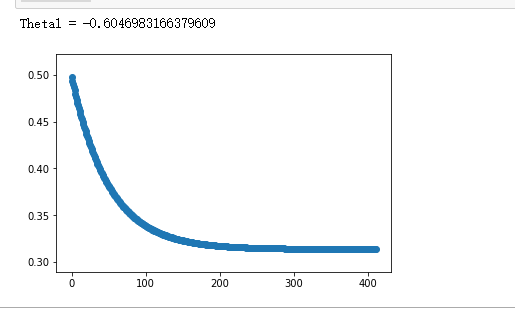展开全文• 一、 案例说明 本节定义一个小汽车的类Car，类中包括车名carname、百公里油耗oilcostper100km、价格price三个属性。然后实现__lt__、__gt__、__le__、__ge__四个方法(这4个方法的用途请见上一节《第8.21节 Python中_...
一、	案例说明
本节定义一个小汽车的类Car，类中包括车名carname、百公里油耗oilcostper100km、价格price三个属性。然后实现__lt__、__gt__、__le__、__ge__四个方法(这4个方法的用途请见上一节《第8.21节 Python中__lt__、__gt__等 “富比较”(“rich comparison”)方法用途探究》(https://blog.csdn.net/LaoYuanPython/article/details/95042104)，为了说明问题，我们将__lt__与__le__的比较逻辑以及__gt__与__ge__的比较逻辑故意弄成了相反，同时重写了__repr__方法以输出格式化的详细信息。然后我们定义两个实例变量来进行大小比较，看执行效果。
二、	案例代码
>>> class Car():
def __init__(self,carname,oilcostper100km, price):
self.carname,self.oilcostper100km,self.price = carname,oilcostper100km, price
def __lt__(self,other):
print("execute __lt__")
return self.price<other.price
def __le__(self,other):
print("execute __le__")
return self.price>other.price
def __gt__(self,other):
print("execute __gt__")
return self.oilcostper100km>other.oilcostper100km

def __ge__(self,other):
print("execute __ge__")
return self.oilcostper100km<other.oilcostper100km

def __repr__(self):  #重写__repr__方法输出repr信息
return  f"('{self.carname}',{self.oilcostper100km},{self.price})"

>>> car1,car2 = Car('爱丽舍',8,10),Car('凯美瑞',7,27)
>>> car1<car2,car1<=car2,car1>car2,car1>=car2
execute __lt__
execute __le__
execute __gt__
execute __ge__
(True, False, True, False)
>>>

三、	案例截屏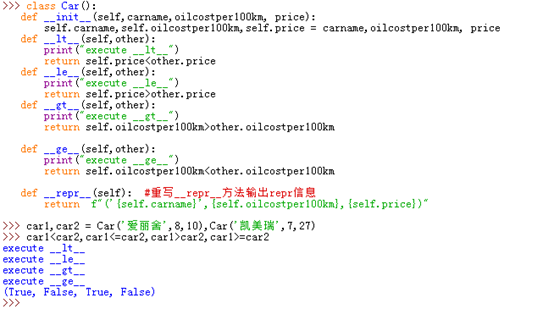四、	案例分析

通过4个比较的输出信息可以得知，<、<=、>、>=分别调用了__lt__、__le__、__gt__、__ge__四个方法;
由于__lt__、__le__使用price进行比较，且真正的比较表达式是相反的两个逻辑，所以二者输出结果也完全相反，同理__gt__、__ge__也是一样，这说明Python自定义类的实例对象比较大小时，对象的大小判断规则是由开发者自己定义的，并没有要求“小于等于”就一定要包含“小于”，二者之间在实现时没有逻辑关系，__gt__与__ge__也如此，同理__gt__与__lt__也无需是相反的结果，最终的逻辑应该根据业务需要进行重写确认；
通过以上案例，我们也知道，通过重写富比较方法，我们完全可以截获对象比较逻辑的调用；
实际的富比较方法还有__eq__和__ne__，这两个方法object类实现了，可以直接继承使用，但也可以重写。

本节参考资料：
1、《第8.21节 Python中__lt__、__gt__等 “富比较”(“rich comparison”)方法用途探究》；
2、《第8.15节 Python重写自定义类的__repr__方法》
老猿Python，跟老猿学Python!
博客地址：https://blog.csdn.net/LaoYuanPython
请大家多多支持，点赞、评论和加关注！谢谢！


展开全文• 上节详细介绍了利用@property装饰器定义属性的语法，本节通过具体案例来进一步说明。 一、 案例说明 本节的案例是定义Rectangle（长方形）类，为了说明问题，除构造函数外，其他方法都只定义了长度属性的操作。包括...
• 1、Python编写的源程序扩展名为（ A）。A. py B. cpp C. doc D. mp42、阅读程序写结果。print("1+1")第1题选A，这个没问题，可是第2题，有初学者掉“坑”里了这就说明对于print打印输出函数不理解，正确...
• 本文实例讲述了Python综合应用名片管理系统。分享给大家供大家参考，具体如下：综合应用已经学习过的知识点：变量流程控制函数模块开发 名片管理系统系统需求程序启动，显示名片管理系统欢迎界面，并显示功能菜单***...
• Scrapy简介Scrapy是用纯Python实现一个为了爬取网站数据、提取结构性数据而编写的应用框架，用途非常广泛。框架的力量，用户只需要定制开发几个模块就可以轻松的实现一个爬虫，用来抓取网页内容以及各种图片，非常之...
• Python运算符重载Python语言提供了运算符重载功能，增强了语言的灵活性，这一点与C++有点类似又有些不同。鉴于它的特殊性，今天就来讨论一下Python运算符重载。Python语言本身提供了很多魔法方法，它的运算符重载...
• 第7.20节 案例详解Python抽象类之真实子类上节介绍了Python抽象基类相关概念，并介绍了抽象基类实现真实子类的步骤和语法，本节结合一个案例进一步详细介绍。一、 案例说明本节定义了图形的抽象基类Shape，要求...
• 这里顺便送大家一套2020最新python入门到高级项目实战视频教程，可以去小编的Python交流.裙 ：七衣衣九七七巴而五（数字的谐音）转换下可以找到了，还可以跟老司机交流讨教！ 本文的文字及图片来源于网络加上自己的...
• 应为知道列表总数，所以偷个懒直接开始循环 for x in range(1,756): print('当前页面: '+ str(x)) get_list(list_url+str(x)) 运行效果： 以上所述是小编给大家介绍的我用Python抓取了7000 多本电子书案例详解整合，...
• 扫描左上角二维码，关注公众账号 数字货币量化投资，回复“Python例子”，获取以下600个Python经典例子源码├─algorithm│ Python用户推荐系统曼哈顿算法实现.py│ NFA引擎,... python du熊学斐波那契实现.py│ p...
• 因此处理数据是核心环节： 下面是python3.7版本下数据处理程序，数据文件以”|”分割，处理数据时程序指明了分隔符。 这里的x=np.loadtxt(dataset...○数据分析报告可以研习案例写作，数据挖掘结果分析根据研习的...
• 1、Python编写的源程序扩展名为（ A）。A. py B. cpp C. doc D. mp42、阅读程序写结果。print("1+1")第1题选A，这个没问题，可是第2题，有初学者掉"坑”里了这就说明对于print打印输出函数不理解，...
• Python程序设计案例课堂第Ⅰ篇 基础知识1 揭开Python神秘面纱1.1 什么是Python1.2 Python的优点和特性1.2.1 Python的优点1.2.2 Python的特点1.3 搭建Python 3的编程环境1.4 运行Python的3种方式1.5 享受安装成果——...
• 主要介绍了Python scrapy爬取小说代码案例详解,文中通过示例代码介绍的非常详细，对大家的学习或者工作具有一定的参考学习价值,需要的朋友可以参考下
• 主要介绍了Python爬虫爬取新闻资讯案例详解,文中通过示例代码介绍的非常详细，对大家的学习或者工作具有一定的参考学习价值,需要的朋友可以参考下
• 前段时间接触了一个批量抠图的模型库，... 到此这篇关于Python实现了电视里的5毛特效的文章就介绍到这了,更多相关Python 5毛特效内容请搜索脚本之家以前的文章或继续浏览下面的相关文章希望大家以后多多支持脚本之家！
• Python爬取网站页面全部内容html # -*- coding: utf-8 -*- # 引入用于爬虫的一个包urllib2 import urllib2 # 引入正则表达式的包 import re def load(url): ''' 对爬虫进行伪装，并爬取一个页面的所有内容 '''...爬虫 html
• 本文实例讲述了Python单例模式。分享给大家供大家参考，具体如下：单例模式：保证一个类仅有一个实例，并提供一个访问他的全局访问点。实现某个类只有一个实例的途径：1，让一个全局变量使得一个对象被访问，但是他...
• 主要介绍了Python面向对象封装操作,结合具体案例形式详细分析了Python面向对象的封装、扩展等操作技巧与相关注意事项,需要的朋友可以参考下
• Python是一门解释型语言，由荷兰的Guido van Rossum在1989年圣诞节期间发明，于1991年公开发布。在设计之初，Python语言被定位在是解释型语言，语法优雅、简单易学、开源、拥有易于扩充开发第三方扩展库。正是这样的......

# python案例详解python 订阅